# The standard quantity per unit defines the ________. price that should be paid for each unit of direct materials. to...

The standard quantity per unit defines the ________.

• price that should be paid for each unit of direct materials.

• total cost of direct materials that should be used for each unit of finished product.

• amount of direct materials that should be used for each unit of finished product including an
allowance for normal inefficiencies, such as scrap and spoilage.

• amount of direct labor-hours that should be used to produce one unit of finished goods.

A flexible budget performance report for variable manufacturing costs shows _______.

• only the activity variances.

• both the activity variances and the spending variances.

• both the revenue variances and the spending variances.

• both the quantity variances and the price variances.

The standard quantity allowed is _______.

• the amount of an input that should have been used to complete the planned output for the period.

• the actual amount of input that was used to complete the planned output for the period.

• the amount of an input that should have been used to complete the actual output for the period.

• the actual amount of input that was used to complete the actual output for the period.

The standard hours allowed is ________.

• the direct labor-hours that should have been used to complete the planned output for the period.

• the direct labor-hours that should have been used to complete the actual output for the period.

• computed by multiplying the standard labor-hours allowed per unit by the planned output for the period.

• computed by multiplying the actual labor-hours per unit by the planned output for the period.

Knowledge Check 01

When computing variable manufacturing overhead variances, the standard rate represents the ________.

• variable portion of the predetermined overhead rate.

• standard hourly pay rate for direct laborers.

• the amount of hours allowed for the actual output.

Assume that direct labor-hours are used as the overhead allocation base. If the direct labor efficiency variance is unfavorable, the variable overhead efficiency variance ________.

• will be favorable.

• will be unfavorable.

• cannot be determined without additional information.convert materials into the finished product.

• will be equal to zero.

The standard quantity per unit defines the amount of direct materials that should be used for each unit of finished product including an allowance for normal inefficiencies, such as scrap and spoilage.

Other answers are incorrect because the standard qty does not define any kind of costs or labor hours. It is the quantity of direct materials.

A flexible budget performance report for variable manufacturing costs shows both the activity variances and the spending variances.

Flexible budget performance reports shows the TOTAL VARIANCE which consists of activity variance plus spending variance.

The standard quantity allowed is the amount of an input that should have been used to complete the actual output for the period

Standard quantity is the standard input that would have produced the actual output.
Suppose 1kg of material X can produce 3 units of Product A [Standard]. 450 units were produced with 145 kg. Here actual input is 145kg which should have been 150 kg. 150 kg is the standard qty allowed

The standard hours allowed is the direct labor-hours that should have been used to complete the actual output for the period.

The logic is similar to standard quantity allowed.

When computing variable manufacturing overhead variances, the standard rate represents the variable portion of the predetermined overhead rate

Assume that direct labor-hours are used as the overhead allocation base. If the direct labor efficiency variance is unfavorable, the variable overhead efficiency variance will be unfavorable

This is because the number of actual hours are more than the budgeted hours for actual output. It will always be unfavorable.

##### Add Answer of: The standard quantity per unit defines the ________. price that should be paid for each unit of direct materials. to...
Similar Homework Help Questions
• ### Direct Material Variances Goldman, Inc. is a manufacturer of lead crystal glasses. The standard direct materials quantit...

Direct Material Variances Goldman, Inc. is a manufacturer of lead crystal glasses. The standard direct materials quantity is 0.7 pounds per glass at the cost of \$0.35 per pound. The actual result for one month’s production of 6,950 glasses was 1.3 pounds per glass, at the cost of \$0.45 per pound. Calculate the direct materials cost variance and the direct materials efficiency variance. Direct Labour Variances Goldman, Inc. manufactures lead crystal glasses. The standard direct labor time is 0.5 hours...

• ### Direct Material Price Variance, Direct Material Quantity Variance

The following data is given for the Taylor Company:Budgeted production 1,000 unitsActual production 980 unitsMaterials:Standard price per lb \$2.00Standard pounds per completed unit 12Actual pounds purchased and used in production 11,800Actual price paid for materials \$23,000Labor:Standard hourly labor rate \$14 per hourStandard hours allowed per completed unit 4.5Actual labor hours worked 4,560Actual total labor costs \$62,928Overhead:Actual and budgeted fixed overhead \$27,000Standard variable overhead rate \$3.50 per standard labor hourOverhead is applied on standard labor hours.The direct material prive variance is?...

• ### Standard Cost per Unit Actual Cost per Unit \$ 3.96 \$ 4.20 12.60 Direct materials: Standard: 1.80 feet at \$2.20 per foot...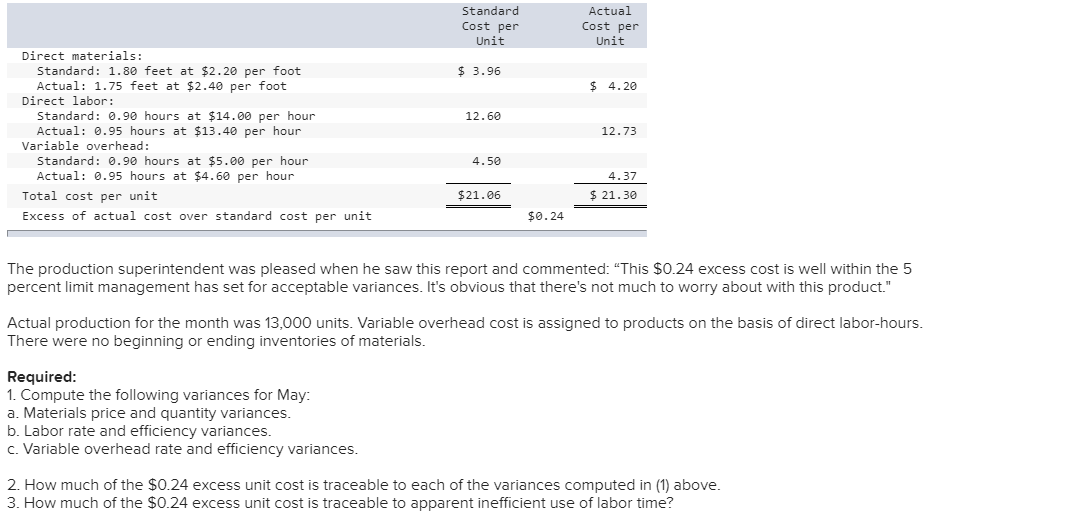Standard Cost per Unit Actual Cost per Unit \$ 3.96 \$ 4.20 12.60 Direct materials: Standard: 1.80 feet at \$2.20 per foot Actual: 1.75 feet at \$2.40 per foot Direct labor: Standard: 0.90 hours at \$14.00 per hour Actual: 0.95 hours at \$13.40 per hour Variable overhead: Standard: 0.90 hours at \$5.00 per hour Actual: 0.95 hours at \$4.60 per hour Total cost per unit Excess of actual cost over standard cost per unit 12.73 4.50 4.37 \$ 21.30 \$21.06...

• ### Trico Company set the following standard unit costs for its single product. Direct materials (30 Ibs. @ \$4.00 per Ib.) \$...

Trico Company set the following standard unit costs for its single product. Direct materials (30 Ibs. @ \$4.00 per Ib.) \$ 120.00 Direct labor (7 hrs. @ \$14 per hr.) 98.00 Factory overhead—Variable (7 hrs. @ \$7 per hr.) 49.00 Factory overhead—Fixed (7 hrs. @ \$11 per hr.) 77.00 Total standard cost \$ 344.00 The predetermined overhead rate is based on a planned operating volume of 80% of the productive capacity of 63,000 units per quarter. The following flexible budget...

• ### The roofing company manufactures shingles. Standard Cost Sheet per shingle 1.5 pounds \$0.07 per pound direct labor Direct materials Asphalt 0.01 hour \$11 per hour Direct labor Variable direct la...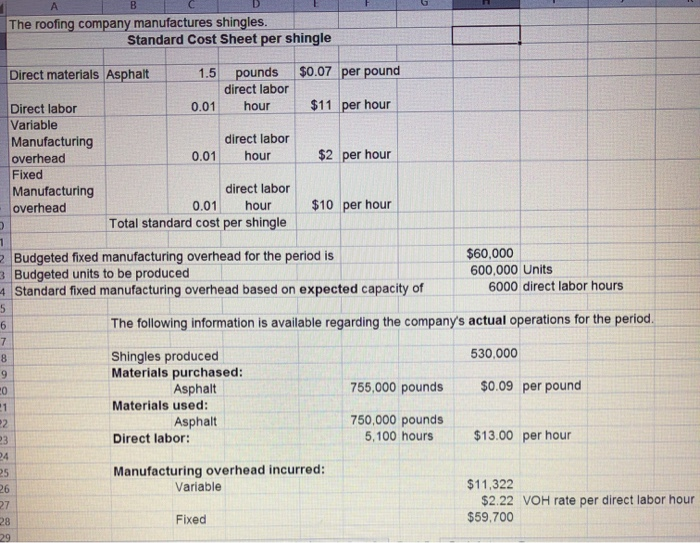The roofing company manufactures shingles. Standard Cost Sheet per shingle 1.5 pounds \$0.07 per pound direct labor Direct materials Asphalt 0.01 hour \$11 per hour Direct labor Variable direct labor Manufacturing \$2 per hour 0.01 hour overhead Fixed direct labor Manufacturing 0.01 hour \$10 per hour overhead Total standard cost per shingle \$60,000 600,000 Units 6000 direct labor hours Budgeted fixed manufacturing overhead for the period is Budgeted units to be produced Standard fixed manufacturing overhead based on expected capacity...

• ### Direct Materials Variances Bellingham Company produces a product that requires 6 standard pounds per unit. The standard...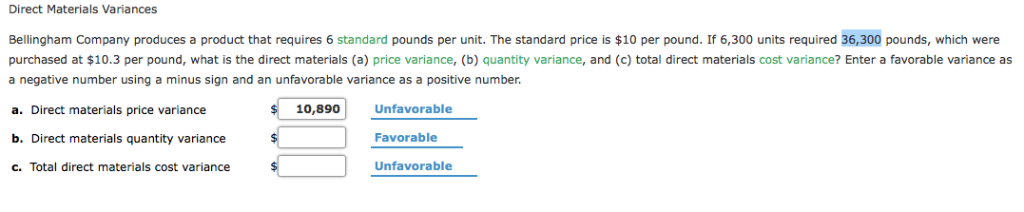Direct Materials Variances Bellingham Company produces a product that requires 6 standard pounds per unit. The standard price is \$10 per pound. If 6,300 units required 36,300 pounds, which were purchased at \$10.3 per pound, what is the direct materials (a) price variance, (b) quantity variance, and (c) total direct materials cost variance? Enter a favorable variance as negative number using a minus sign and an unfavorable variance as a positive number. 10,890 Unfavorable a. Direct materials price variance b....

• ### Hutto Corp. has set the following standard direct materials and direct labor costs per unit for the product it manufact...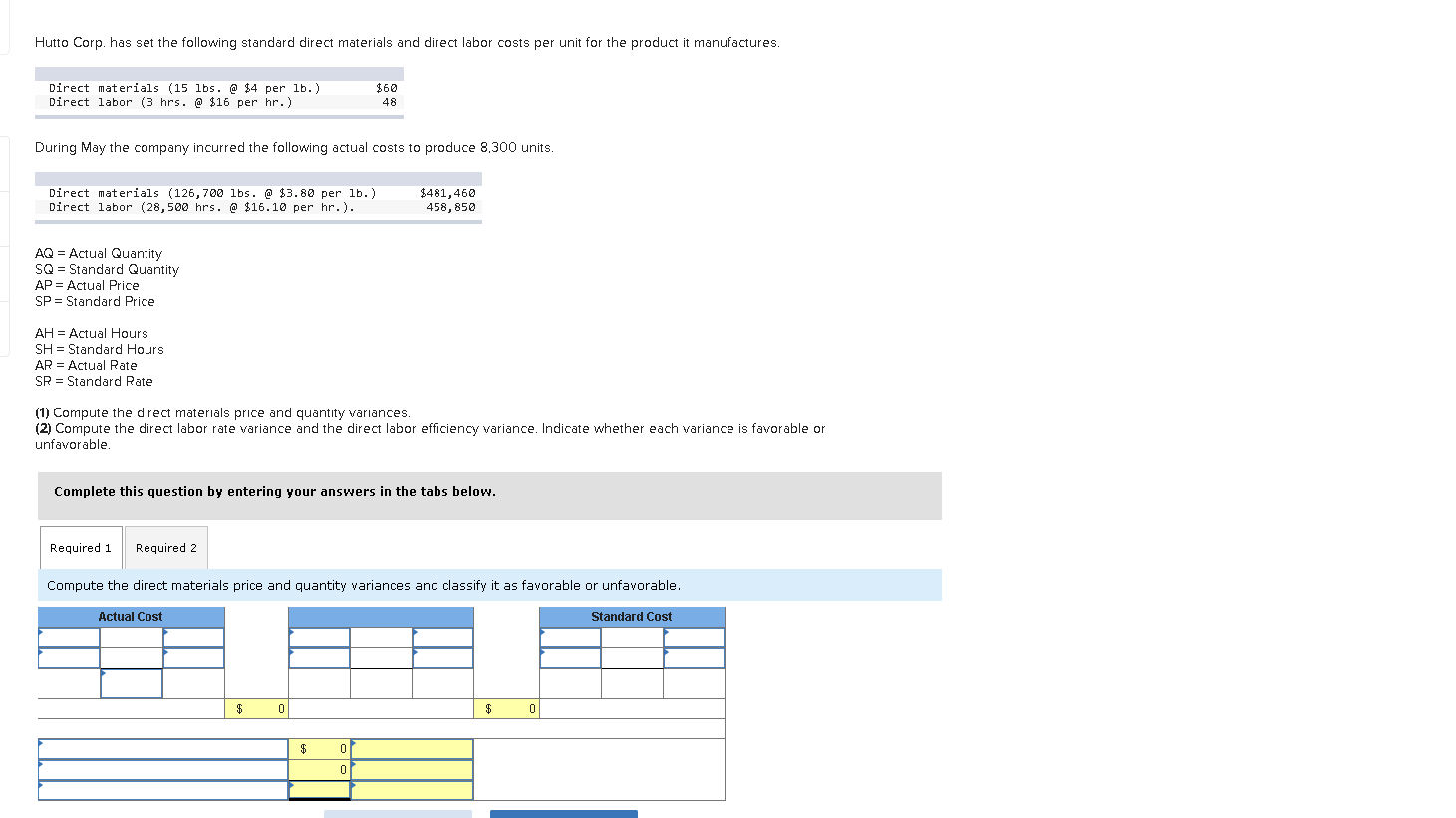Hutto Corp. has set the following standard direct materials and direct labor costs per unit for the product it manufactures. Direct materials (15 lbs. @ \$4 per lb.) Direct labor (3 hrs. @ \$16 per hr.) \$60 48 During May the company incurred the following actual costs to produce 8,300 units. Direct materials (126,700 lbs. @ \$3.80 per lb.) Direct labor (28,500 hrs. @ \$16.10 per hr.). \$481,460 458,850 AQ = Actual Quantity SQ = Standard Quantity AP = Actual...

• ### Hutto Corp. has set the following standard direct materials and direct labor costs per unit for...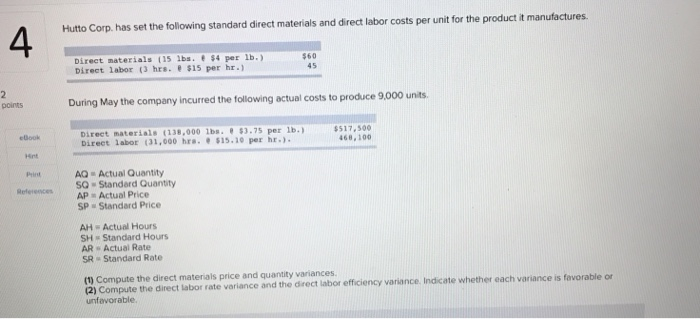Hutto Corp. has set the following standard direct materials and direct labor costs per unit for the product it manufactures. Direct materials (15 lbs. \$4 per lb.) Direct labor ( hrs. \$15 per hr.) Sosis 15.) 588 points During May the company incurred the following actual costs to produce 9,000 units. Direct materiale 18.000 lbs. ).75 per 1.) Direct labor 31.000 hrs. 515.10 per hr.). \$517.500 468,100 AQ - Actual Quantity SQ - Standard Quantity AP Actual Price SP Standard...

• ### Direct materials price variance and quantity variance question.

TenPro reports the following on one of its products.Direct materials standard (4 lbs. @ \$2/lb.) \$ 8 per finished unitActual direct materials used 150,000 lbs.Actual finished units produced 30,000 unitsActual cost of direct materials used \$ 267,500Compute the direct materials price and quantity variances.It would be most helpful to explain the steps vice just the answer. Thanks!

• ### Antuan Company set the following standard costs for one unit of its product. Direct materials (5.0...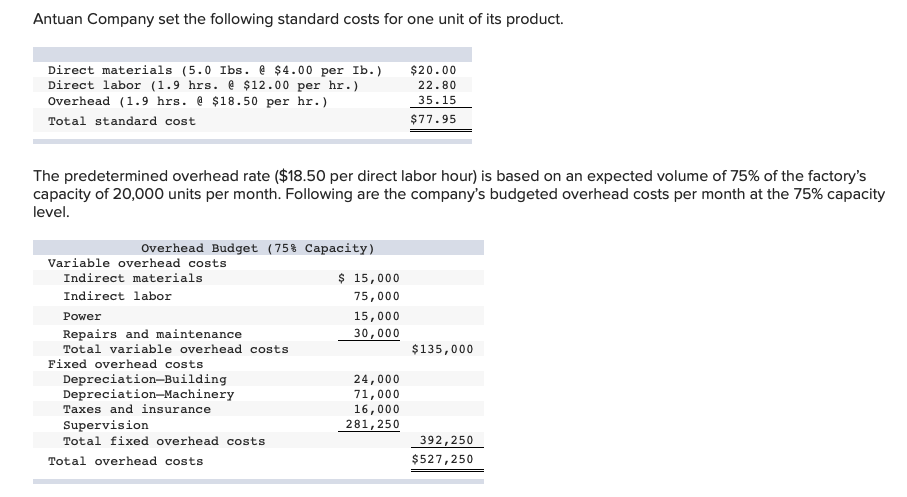Antuan Company set the following standard costs for one unit of its product. Direct materials (5.0 Ibs. \$4.00 per Ib.) Direct labor (1.9 hrs. \$12.00 per hr.) Overhead (1.9 hrs. \$18.50 per hr.) \$20.00 22.80 35.15 \$77.95 Total standard cost The predetermined overhead rate (\$18.50 per direct labor hour) is based on an expected volume of 75% of the factory's capacity of 20,000 units per month. Following are the company's budgeted overhead costs per month at the 75% capacity level....

Need Online Homework Help?